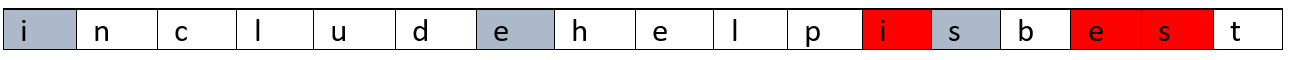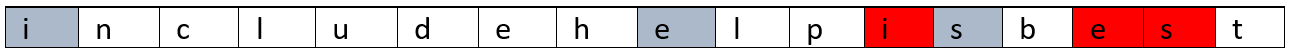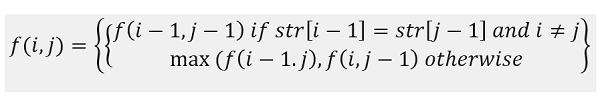# Longest Repeating Subsequence

Longest Repeating Subsequence: Here, we are going to learn about the solution of Longest Repeating Subsequence – which is an interview coding questions featured in any rounds of top companies.
Submitted by Radib Kar, on February 21, 2020

Description:

This question has been featured in interview rounds of Amazon.

Problem statement:

Given string str, find the length of the longest repeating subsequence such that the two subsequences don't have the same character in the same position, i.e., any ith character in the two sub-sequences shouldn't have the same index in its original string.

```    Let the string be,
Str="includehelpisbest"

Output will be:
Longest repeating sub-sequence length is 3
The longest repeating sub-sequence is:"ies"
```The output is given above where the repeating sub-sequences are in two different colours. One more repeating subs-sequence can be,Solution Approach:

The problem can be solved in similar way as longest Common subsequence problem, where input to LCS() would be String str both the case. That is

```    LRS(str) = LCS(str,str) which some constraints
```

Let's discuss the recursive approach first.

Let,

```
l = Length of the  string,str
f(l,l) = Longest repeating subsequence length for string length l
```

Now,

Think of the following example,

Say the string is: x1x2...xl

Say,

xi==xj and i≠j (this is the constraint what we discussed above)

Then obviously we need to find LCS for the remaining part of string (x1x2...xi-1, x1x2...xj-1) and then add 1 for this valid character match (repeating character match),

Else

Maximum of two case,

1. LCS of the string leaving character xi,x1x2...xi-1 and string x1x2...xj.
2. LCS of the string xl1, x1x2...xi and second string leaving character xj,x1x2...xj-1

Now, we need to recur down to 0. So,Where base cases are,

```    f(0,i)=0 for 0≤i≤l
f(i,0)=0 for 0≤i≤ l
```

If you generate this recursion tree, it will generate many overlapping sub-problems and thus, we need to reduce the re-computing. That's why we need to convert it into dynamic programming where we will store the output of the sub-problems and we will use it to compute bigger sub-problems.

Converting to Dynamic programming:

```    1)  Initialize dp[l+1][l+1]  to 0
2)  Convert the base case of recursion:
for i=0 to l
dp[i]=0;
for i=0 to l
dp[i]=0;

3)  Fill the DP table as per recursion.
for i=1 to l    //i be the subproblem length for first string of LCS
for j=1 to l //j be the subproblem length for second string of LCS
if(i≠j and str1[i-1]==str2[j-1]) //xi==xj  and i≠j
dp[i][j]=dp[i-1][j-1]+1;
else
dp[i][j]=max(dp[i-1][j],dp[i][j-1]);
end for
end for
4)  The final output will be dp[l][l]
```

C++ Implementation:

```#include <bits/stdc++.h>
using namespace std;

void print(vector<int> a, int n)
{
for (int i = 0; i < n; i++)
cout << a[i] << " ";
cout << endl;
}

int max(int a, int b)
{
return (a > b) ? a : b;
}

int LRS(string str1, string str2)
{
int l = str1.length();
int dp[l + 1][l + 1];

//base case
for (int i = 0; i <= l; i++)
dp[i] = 0;

for (int i = 0; i <= l; i++)
dp[i] = 0;

//fill up
for (int i = 1; i <= l; i++) {
for (int j = 1; j <= l; j++) {
if (i != j && str1[i - 1] == str2[j - 1])
dp[i][j] = dp[i - 1][j - 1] + 1;
else
dp[i][j] = max(dp[i - 1][j], dp[i][j - 1]);
}
}

return dp[l][l];
}

int main()
{
string str;

cout << "Enter the string:\n";
cin >> str;
cout << "Longest repeating sub-sequence length: " << LRS(str, str) << endl;

return 0;
}
```

Output

```Enter the string:
includehelpisbest
Longest repeating sub-sequence length: 3
```